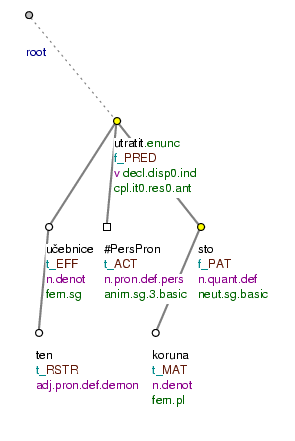### 4.1. Topic-focus articulation and the lexical content of an expression

The lexical content (t-lemma) of a modification can be helpful in the annotation of contextual boundness. Certain lexical units are usually contextually bound and certain other lexical units tend to be contextually non-bound.

There are the following tendencies in the relationship between the topic-focus articulation and the lexical content of an expression:

• lexical units denoting something indefinite, unknown (indefinite pronouns, numerals and adverbs; e.g.: někdo (=somebody), něco (=something), jednou (=once), nějaký (=some) etc.) tend to be contextually non-bound.

Nodes representing such lexical units are usually assigned the `tfa` value `f` .

• deictic expressions (i.e. some pronouns, pronominal adverbs such as tam (=there), tady (=here), tudy (=through_here), tehdy (=then) and others) are mostly contextually bound (both contrastive and non-contrastive). These are lexical units referring to facts as if they were known and so connect them into the situational context or into the context of shared knowledge. Therefore they include also lexical units deducible from known facts (e.g.: včera (=yesterday), zítra (=tomorrow), pozítří (=day_after_tomorrow)). In this quite large set there are expressions with a stronger tendency (demonstrative pronouns) and expressions with a weaker tendency (possessive pronouns) towards contextual boundness.

The value `t` in the attribute `tfa` is primary for nodes representing such expressions; when they are used contrastively they can have also the value `c`. However, these expressions can constitute the focus proper (in case they carry the intonation centre) and have the value `f`.

Examples:

Mně [`tfa`=`c`] ani tobě [`tfa`=`c`] se to [`tfa`=`t`] nestane [`tfa`=`f`] (= To_me nor to_you _ it will_not_happen.)

Ten [`tfa`=`t`] pes [`tfa`=`t`] je [`tfa`=`t`/`f`] MŮJ [`tfa`=`f`] (=lit. The dog is mine.)

• nouns governing numerals functioning as attributes tend towards (non-contrastive) contextual boundness (see Section 10.1.1, "Numerals with the role of an attribute (`RSTR`)"). These nodes usually are names of various units, which are commonly known and are part of the commonly shared knowledge (they can be absent in the surface structure of the sentence), or they are nodes repeated from the context which are further specified by the numerals.

The name of the counted object (the noun governing the numeral in the position of a modification with the functor `RSTR`) can also constitute a quasi-focus (see Section 3.1.2, "Quasi-focus").

Example:

Utratil [`tfa`=`f`] za tu [`tfa`=`t`] učebnici [`tfa`=`t`] sto [`tfa`=`f`] korun [`tfa`=`t`] (=lit. (He) spent for that textbook one_hundred crowns.) Fig. 10.13

NB! Most lexical units that have a tendency towards (non-contrastive) contextual boundness can constitute also the focus proper (see Section 3.1.1, "Focus proper") or form a contrastive contextually bound expression. These two positions can be occupied by almost any lexical unit. It is therefore necessary to distinguish them from other positions where the expressions are (non-contrastive) contextually bound due to their lexical content or syntactic function.

Figure 10.13. Topic-focus articulation and the lexical content of an expressionUtratil za tu učebnici sto korun. (=lit. (He) spent for that textbook one_hundred crowns.)

Exceptional cases - personal pronouns. Also the form of a modification can be helpful when annotating contextual boundness. The form used is substantial in the case of personal pronouns. In Czech, for personal pronouns there are two sets of forms for certain cases - strong (jeho (=he.ACC), jemu (=he.DAT), sebe (=oneself.ACC), sobě (=oneself.DAT), tobě (=you.DAT), tebe (=you.ACC)) and weak (ho (=he.ACC), mu (=he.ACC), se (=oneself.ACC), si (=oneself.DAT), tě (=you.ACC), ti (=you.DAT)).

If both strong and weak forms of a pronoun can be used, the actual form is usually motivated by the contextual boundness of the pronoun:

• weak forms appear in these positions only when the pronoun is (non-contrastive) contextually bound. Nodes representing the weak forms of pronouns (such as tě (=you.ACC), ti (=you.DAT), ho (=he.ACC), mu (=he.DAT), mi (=I.DAT) and often also neutral forms mě (=I.ACC, I.GEN), mně (=I.DAT, I.LOC), ji (=she.ACC), jí (=she.DAT), jim (=they.ACC), jej (=he.ACC), nás (we.ACC) etc.) are assigned the `tfa` value `t`.

• nodes representing the strong forms of pronouns (such as tebe (=you.ACC) , jemu (=he.DAT) etc.) and neutral forms of pronouns are assigned the value `f` if they are the bearers of the intonation centre (see Section 1.2.1, "Intonation centre"), or they are assigned the value `c` if they are used contrastively.

NB! After prepositions and in coordination, personal pronouns always take the strong form, so we cannot use their form to determine their contextual boundness. In such positions nodes representing the strong forms of pronouns get the `tfa` value `t`.

Examples:

Pro tebe [`tfa`=`c`] to přinesu ZÍTRA. (=lit. To you it.ACC (I) will_bring tomorrow.)

Zítra to pro tebe [`tfa`=`t`] PŘINESU. (=lit. Tomorrow it.ACC to you (I) will_bring.)

Zítra to přinesu PRO TEBE [`tfa`=`f`] (=lit. Tomorrow it.ACC (I) will_bring to you.)

ZÍTRA to pro tebe [`tfa`=`t`] přinesu. (=lit. Tomorrow it.ACC to you (I) will_bring.)

Jemu [`tfa`=`c`] a tobě [`tfa`=`c`] to přinesu ZÍTRA. (=lit. To_him and to_you it.ACC (I) will_bring tomorrow.)

Zítra to jemu [`tfa`=`t`] a tobě [`tfa`=`t`] určitě PŘINESU. (=lit. Tomorrow it.ACC to_him and to_you by_all_means (I) will_bring.)